Tuesday, 23 November 2010

Could William Blake have been right, could everything be an infinity?

~

A quote from William Blake “If the door of perception were cleansed everything would appear to man as it is, infinite”. Could William Blake have been right, could everything be an infinity?
A link to the video “Could our Universe be an infinity” This video explains how we can have a potential infinity of probabilities at every degree and angle of creation. This is because of the probabilistic nature of the wave particle duality of light.

In modern physics there is only a mathematical interpretation of the wave particle duality of light called the quantum wave particle function.

But in this theory the quantum wave particle function is a physical process continuously collapsing and reforming that forms the forward passage of time photon by photon or moment by moment.

The forward momentum of light forms the forward passage of time and geometry of spacetime.
~The quantum wave particle function is a difficult concept to understand because it expands as a volume of space in the shape of an inverse sphere.

The reason for this is that we are part of the process or within the process.
It is like being within a room that is square in shape the room will be an inverse square because we are on the inside look out.

~Therefore we have an inverse sphere that will expand out from zero in all directions along the x and y axis as part of an infinite process.

The spherical shape of the quantum wave particle function can be seen in the equations of quantum physics (∆×∆p×≥h/4π) in the form of 4 π representing a sphere.

In this theory π is more than just the ratio of any circle’s circumference to its diameter more than a static universal constant. The irrational number π that forms the infinite series 3.14159265979… represents the geometry of a dynamic process of symmetry forming and breaking forming the time continuum and the geometry we see in everyday life.

This can be mathematically explained by what Cantor called ‘the continuum hypotheses’. Cantor discovered a structure to infinity and that there are more numbers between zero and one than there are whole numbers.

He found that we can build greater and greater infinities from zero up and that the further you move away from zero the less infinities you have.

This theory can give us a physical starting point to any infinite series. We can choose when and where to collapse the quantum wave particle function forming new particles in space and new moments in time.

This will form a new wave function of future potential that will expand out from zero in all directions forming an infinity of possibilities.

The forward momentum of light or EMR is continuously collapsing and reforming. This forms a universal fractal branching process that forms the geometry of spacetime and the arrow of time itself.

Therefore Heisenberg’s Uncertainty Principle is the same uncertainty that we have with any future event.
~

Sunday, 14 November 2010

~

This post will put forward the view that Zeno’s paradoxes represent something fundamentally wrong with our understanding of the structure of space and time.

This forms a problem that runs through the whole of human mathematics.

That starts with the discovery of irrational numbers in ancient Greece and forms infinities at the base of Newton and Leibniz calculus.

It can also be seen at the heart of Cantor’s set theory and also as a paradox in his continuum hypothesis.

Quantum electrodynamics also suffers from the problem of infinities that has not properly been solved.

There is also the measurement problem of quantum physics that has no logical explanation.

All this can only be explained by a fundamental change in our concept of space and time.

Zeno’s paradox can be expressed in many ways but for the purpose of this post it is best to think of dropping an object and measuring the time it takes to reach the ground.

We can always half the length of time the object takes to reach the ground and by doing this we will form an infinite series therefore the object will never reach the ground.

Zeno rejected the idea of infinity and so he had a paradox and believed that moving and changing to be an illusion and that only what ‘was’ mattered!

But the theory I am now going explain does not reject infinity but explains it as a universal process that forms the arrow of time and geometry of spacetime. (This does not mean that Zeno was wrong)

In this theory the forward passage of time is formed by the forward motion or momentum of light (EMR) forming the geometry of spacetime.
The probabilistic nature of the wave particle duality of light forms the flow of time itself. This is explained by Schrodinger equation that represents the quantum wave particle function.

Therefore Heisenberg’s Uncertainty Principle is the same uncertainty that we have with any future event and represents potential future possibilities.

The answer to the problem of infinity is that it only has the potential probability to exist. Aristotle was the first to introduce the idea of something being potentially infinite.

In this theory we have a potential infinity of probabilities at every degree and angle of spacetime because one thing after another is always coming into existence as part of the time continuum.
~Geory Cantor
~

This can be explained mathematically by what Cantor called ‘the continuum hypothesis’ because it deals with the continuum of numbers between 0 and 1.

Cantor believed that we have more than one kind of infinity. There are more numbers between 0 and 1 than there are integers, more than infinity.

Set theory lies at the heart of mathematics but Cantor’ set theory relies on there being something called the empty set a set of nothingness.

One of the axioms (principles) of set theory is that we have a choice of choosing one member of any non-empty subset of the set.

This has caused a problem proving the continuum hypothesis. Because there is no explanation of human interaction, how is the choice to be made?

In this theory we have a physical starting point to any infinite series. We can choose when and where to collapse the quantum wave particle function forming new particles in space and new moments in time.

This will form a new wave function of future potential that will expand out from zero in all directions along the x and y axis forming part of an infinite process.

Just has Cantor could mathematically build up a never-ending series of larger and larger infinities this theory can also do the same in the physical world. This fits in with Pythagoras’ most central belief that reality has a mathematical base.

Therefore the infinities in the mathematics of Quantum electrodynamics are not a problem; they represent the continuum of time itself that is infinite and there is no need for the process call renormalization.
~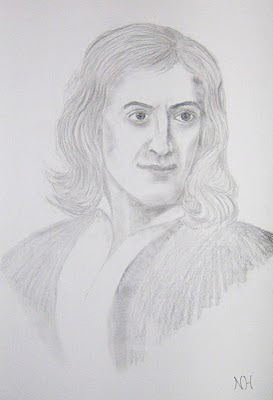Sir Isaac Newton

~

Newton believed the Universe to be a true infinity. In the creation of calculus Newton thought in terms of motion of fluidity not in terms of the infinitely small.

This continuous process of change can be seen has a universal process of symmetry forming and breaking that forms fractal self similarities

Fractals, chaos theory shows that it is possible to have infinity in a finite world (Koch curve) all we need is a way of dividing space up into infinitely small portions.

This theory explains a process of how spacetime can be continuously formed that can always be dived up into infinitely small portions (mathematical infinity).
~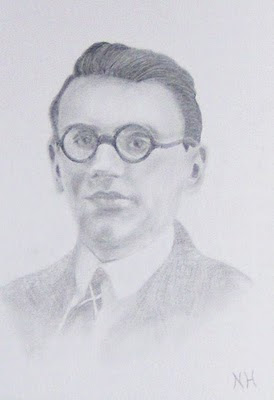Kurt Godel

~

This also explains Kurt Gödel, Incompleteness Theorem some problems in mathematics can’t be solved no matter how you approach the problem.
Therefore we have the Measurement Problem and can never know the position and momentum of a quantum particle at the same time. This is because in the world something is in existence or it has the potential to exist, but never both.

In this theory mathematical infinity, physical infinity and absolute infinity are all one and the same.

~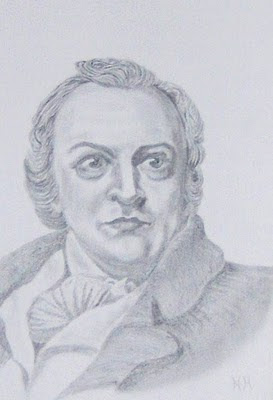“If the door of perception were cleansed everything would appear to man as it is, infinite”.
William Blake 1757…

~

Sunday, 7 November 2010

Could a lack of imagination have led to the Copenhagen interpretation of Quantum Mechanics?

This post will put forward the idea that by explaining the Universe (reality) as a continuum that is in a continuous process of creation, we can also explain Quantum mechanics as part of an objective reality.
~
In this theory (Quantum Atom Theory) this universal and continuous process is formed by the quantum wave particle function.
~
That is continuously collapsing and reforming creating new photon particles in space and new moments in time.
~
In this theory the forward momentum of light forms the forward passage of time or arrow of time photon by photon or moment by moment.
~
Therefore Heisenberg’s Uncertainty Principle is the same uncertainty that we have with any future event. The Heisenberg’s Uncertainty Principle does not work in the same way forwards and backwards in time.
~
We can always calculate backwards and find the position and momentum of a quantum particle in the past. This totally fits in with our everyday experience of the nature of time, moving from a known past into an uncertain future.

~

Wednesday, 3 November 2010

Is the observer effect true or false?

Some people say that the paradoxes of quantum mechanics can only be explained if it is linked to the consciousness of the observer in the so called “observer effect”. But this theory will put forward the opposite idea that a deeper understanding of the physics of quantum mechanics will give us a greater understanding of how consciousness works.
~We can see in the Two Slit Experiment that it is only when a detector is turned on that the interference pattern collapses. In other words only when light comes into physical contact with something does it collapse into photons or particles. When the detector is turned off the interference pattern will reform.
~It is the conscious idea that leads to the physical action but it is the action itself that collapses the wave function of quantum physics.
~
Only an understanding of the process that forms the passage of time and geometry of spacetime will explain quantum mechanics.
~In this theory each new moment in time is formed by a single photon electron coupling creating a wave-function of future possibilities.
~
The inward absorption and outward emission of light or EMR forms the arrow of time and geometry of spacetime. We live in a Universe of infinite possibilities (Aristotle’s potential infinity) because of the Probabilistic nature of light.
~
On the level of everyday objects the observer will see an infinite number of photon electron couplings, forming a temporary image of the Universe, photon by photon or moment by moment.
~
Each new photon electron coupling will have its own position in three dimensional space and a position in time as part of the passage of time this is why everything is relative too everything else and we have Einstein’s general theory of relativity.
~
In this theory we form are own quantum particles, virtual particles only manifest themselves when something comes in contact with the quantum vacuum.

~The atoms bond together and react with the wave particle duality of the light in unison forming their own future spacetime geometry we see and feel this as the free-will of everyday life.
~This speculative diagram highlights the profound symmetry between matter and antimatter, positive and negative charge.
~It is the inward absorption and outward emission of light or EMR that forms matter antimatter annihilation forming positive and negative charge that in turn forms electromagnetic fields.
~Because of this process we have a potential infinity of future possibilities at every point in space and at every moment in time that can spiral out forming its own spacetime geometry. We have a continuous process of symmetry forming and breaking that formed a geometrical therefore mathematical base for the evolution of life.
~This geometry that predated the evolution and gave life a mathematical base can still be seen today in the curvature and line symmetry of the individual life form.
~

Therefore we never have total chaos and everything is based on the same universal geometry and symmetry.

~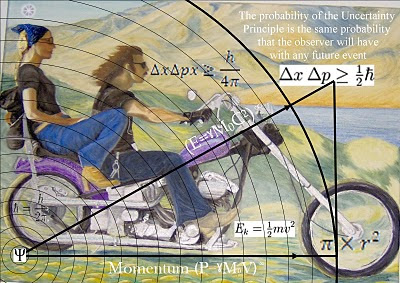The continuous random breaking of this symmetry can be seen as Heisenberg’s Uncertainty Principle that is the same uncertainty that we have with any future event.
~This is because the quantum wave-particle function that is explained mathematically by Schrödinger’s wave equation represents the forward passage of time itself photon by photon or moment by moment.

~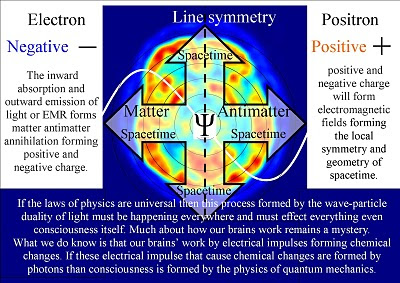The laws of physics are universal therefore this process formed by the wave-particle duality of light must be happening everywhere and must effect everything even consciousness itself.
~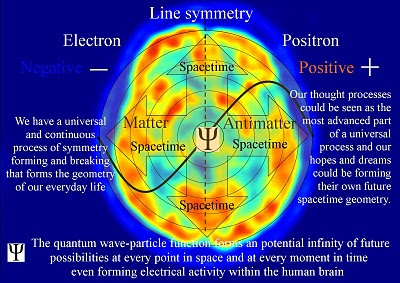Despite rapid scientific progress, much about how our brains work remains a mystery. What we do know is that our brains’ work by electrical impulses forming chemical changes, this electrical activity forms electromagnetic fields.
If these electrical impulse that cause chemical changes are formed by photons than consciousness is formed by the physics of quantum mechanics.
~

Therefore our thought processes could be seen as the most advanced part of a universal process and our hopes and dreams could be forming their own potential future spacetime geometry.
~